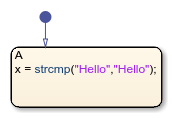strcmp

Compare strings

Description

example

tf = strcmp(s1,s2) compares strings s1 and s2.

When using MATLAB® as the action language, strcmp returns 1 (true) if the two strings are identical. Otherwise, strcmp returns 0 (false).

When using C as the action language, strcmp returns 0 if the two strings are identical. Otherwise, strcmp returns a nonzero integer where:

• The sign of the output value depends on the lexicographic order of the input strings s1 and s2.

• The magnitude of the output value depends on the compiler that you use. This value can differ in simulation and generated code.

Strings are considered identical when they have the same size and content. strcmp is case sensitive.

example

tf = strcmp(s1,s2,n) returns 0 if the first n characters in s1 and s2 are identical in charts that use C as the action language. For this functionality when using MATLAB as the action language, see strncmp.

Examples

expand all

Return a value of 1(true) if the strings are equal.

tf = strcmp("abc","abc");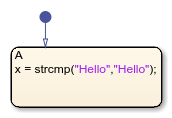Return a 0(false) value if the strings are not equal.

tf = strcmp("abc","abcd");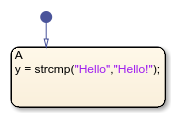You can compare and sort string with relational operators.

Use == to determine which elements of two string are equal. This is an alternative way to execute strcmp("abc", "abc") == 1.

"abc" == "abc"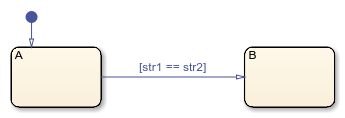Use ~= to determine which elements of two string are not equal. This is an alternative way to execute strcmp("abc", "abc") ~= 1.

"abc" ~= "abc"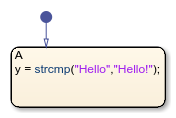Return a value of 0 if the strings are equal.

tf = strcmp("abc","abc");Return a nonzero value if the strings are not equal.

tf = strcmp("abc","abcd");Use == to determine which elements of two strings are equal. This is an alternative way to execute strcmp("abc", "abc") == 1.

"abc" == "abc"Use ~= to determine which elements of two strings are not equal. This is an alternative way to execute strcmp("abc", "abc") != 1.

"abc" != "abc"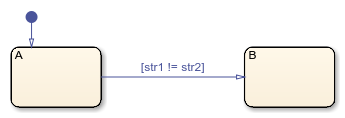Return a value of 0 if the substrings are equal in charts that use C as the action language. To use this functionality when using MATLAB as the action language, see strncmp.

tf = strcmp("abc","abcd",3);